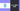# Golang - What is epoch?

04 Apr, 2022, 3 min read

# What is Epoch?

Epoch is a point in time chosen as the reference point. It helps in measuring other time values with respect to the Epoch value. For instance, if you are considering Jan 1, 1970 as an epoch value, then any time after that point can be identified with a single long number. So a number like “86400” can be interpreted as the number of seconds passed after that epoch reference point and it is “Jan 2, 1970 00:00:00 UTC”

Epoch value in Computing depends on the computer system in which it is calculated. It is calculated in terms of seconds or clock cycle (tick) which has elapsed after the initial epoch reference point.

## Why is Epoch useful?

It is easy to calculate the difference between two timestamps using epoch values. Since both the timestamps can be represented in a single numerical value, the difference between the two numerical values will give the time difference.

Let’s look at an example

Date Epoch
Monday, 4 April 2022 13:17:16 1649078236
Saturday, 2 April 2022 06:17:16 1648880236

Time difference = 198000 seconds

Converting the seconds to days = 2 Days 7 Hours

## Epoch values of different operation system

Below table represents the epoch value based on the operating system (OS)

Operating System Epoch
Unix January 1, 1970
Windows January 1, 1601
Mac OS January 1, 1904
POSIX January 1, 1970

Note: Maximum value which can be stored in the timestamp represented in epoch format also depends on the word length (number of bits) of the operating system. Unix system with a word length of 32-bit will reach the maximum value on January 19, 2038.

## How to convert time into epoch value in Go?

You can use the `time` package in Go to get the current time in epoch representation.

``````package main

import (
"fmt"
"time"
)

func main() {

currentTime := time.Now()

// Epoch time in Seconds
fmt.Println(currentTime.Unix())

// Epoch time in Milliseconds
fmt.Println(currentTime.UnixMilli())
}``````

Output

``````1649080363
1649080363834``````

## How to convert epoch value to time in Go?

You can use the same `time` package in go to convert the epoch value to time.

### Syntax of time.Unix()

``func Unix(seconds int64, nanoseconds int64) Time``

The first parameter is the second value if you have the epoch value in seconds. Pass 0 if the value is in nanoseconds

The second parameter is the nanosecond value. Pass 0 if you have epoch in seconds

### Example of time.Unix()

``````package main

import (
"fmt"
"time"
)

func main() {
// Convert epoch in seconds to time
fmt.Println(time.Unix(1649080363, 0))
}``````

Output

``2022-04-04 13:52:43 +0000 UTC``

## Table for the time period and epoch value

Below is the table if you want to convert the epoch value to the time period for calculation

Time period Epoch value
1 minute 60
1 hour 3,600
1 day 86,400
1 month 2,419,200
1 year 29,030,400